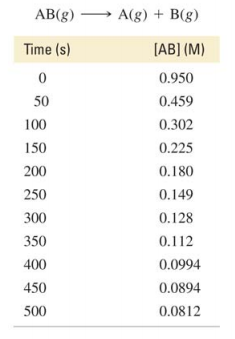# Problem: The data below show the concentration of AB versus time for this reaction: Determine the order of the reaction and the value of the rate constant. Predict the concentration of AB at 25 s.

###### FREE Expert Solution

For this problem, we will need to generate a set of graphs to answer this question. The graph that would generate a straight line is the order of the reaction.

The integrated rate law for a zeroth-order reaction is as follows:

$\overline{){\mathbf{\left[}\mathbf{A}\mathbf{\right]}}_{{\mathbf{t}}}{\mathbf{=}}{\mathbf{-}}{\mathbf{kt}}{\mathbf{+}}{{\mathbf{\left[}}{\mathbf{A}}{\mathbf{\right]}}}_{{\mathbf{0}}}}$

y    =    mx   +  b

where:

[A]t = concentration at time t
k = rate constant
t = time
[A]0 = initial concentration

Graph time vs. [AB]

82% (196 ratings)###### Problem Details

The data below show the concentration of AB versus time for this reaction:

Determine the order of the reaction and the value of the rate constant. Predict the concentration of AB at 25 s.Frequently Asked Questions

What scientific concept do you need to know in order to solve this problem?

Our tutors have indicated that to solve this problem you will need to apply the Rate Law concept. You can view video lessons to learn Rate Law. Or if you need more Rate Law practice, you can also practice Rate Law practice problems.

What textbook is this problem found in?

Our data indicates that this problem or a close variation was asked in Chemistry: A Molecular Approach - Tro 2nd Edition. You can also practice Chemistry: A Molecular Approach - Tro 2nd Edition practice problems.